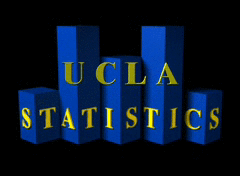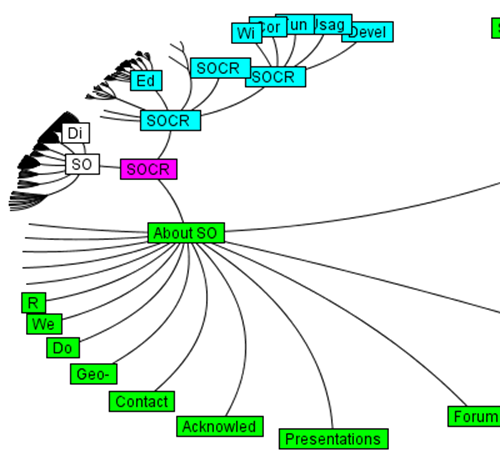SOCR News, Events, Announcements 2010 SOCR Continuing Education Workshop Probability & Statistics EBook It's online, therefore it exists! RSSAdvanced Placement (AP) Statistics Course Overview

The following AP Statistics topics are derived from the CollegeBoard's guideline. These topics are divided into four major themes: exploratory analysis (20–30 percent of the exam), planning and conducting a study (10–15 percent of the exam), probability (20–30 percent of the exam), and statistical inference (30–40 percent of the exam).

 Exploring Data: Describing patterns and departures from patterns. Exploratory analysis of data makes use of graphical and numerical techniques to study patterns and departures from patterns. Emphasis should be placed on interpreting information from graphical and numerical displays and summaries. Constructing and interpreting graphical displays of distributions of univariate data (dotplot, stemplot, histogram, cumulative frequency plot) Center and spread Clusters and gaps Outliers and other unusual features Shape Constructing and interpreting graphical displays of distributions of univariate data (dotplot, stemplot, histogram, cumulative frequency plot) Center and spread Clusters and gaps Outliers and other unusual features Shape Summarizing distributions of univariate data Measuring center: median, mean Measuring spread: range, interquartile range, standard deviation Measuring position: quartiles, percentiles, standardized scores (z-scores) Using boxplots The effect of changing units on summary measures  Comparing distributions of univariate data (dotplots, back-to-back stemplots, parallel boxplots) Comparing center and spread: within group, between group variation Comparing clusters and gaps Comparing outliers and other unusual features Comparing shapes Exploring bivariate data Analyzing patterns in scatterplots Correlation and linearity Least-squares regression line Residual plots, outliers, and influential points Transformations to achieve linearity: logarithmic and power transformations Exploring categorical data Frequency tables and bar charts Marginal and joint frequencies for two-way tables Conditional relative frequencies and association Comparing distributions using bar charts Sampling and Experimentation: Planning and conducting a study: Data must be collected according to a well-developed plan if valid information on a conjecture is to be obtained. This plan includes clarifying the question and deciding upon a method of data collection and analysis. Overview of methods of data collection Census Sample survey Experiment Observational study Planning and conducting surveys Characteristics of a well-designed and well-conducted survey Populations, samples, and random selection Sources of bias in sampling and surveys Sampling methods, including simple random sampling, stratified random sampling, and cluster sampling Planning and conducting experiments Characteristics of a well-designed and well-conducted experiment Treatments, control groups, experimental units, random assignments, and replication Sources of bias and confounding, including placebo effect and blinding Completely randomized design Randomized block design, including matched pairs design Generalizability of results and types of conclusions that can be drawn from observational studies, experiments, and surveys Anticipating Patterns: Exploring random phenomena using probability and simulation: Probability is the tool used for anticipating what the distribution of data should look like under a given model. Probability Interpreting probability, including long-run relative frequency interpretation “Law of Large Numbers” concept Addition rule, multiplication rule, conditional probability, and independence Discrete random variables and their probability distributions, including binomial and geometric Simulation of random behavior and probability distributions Mean (expected value) and standard deviation of a random variable, and linear transformation of a random variable Combining independent random variables Notion of independence versus dependence Mean and standard deviation for sums and differences of independent random variables The normal distribution Sampling distributions Statistical Inference: Estimating population parameters and testing hypotheses: Statistical inference guides the selection of appropriate models. (point estimators and confidence intervals) Estimating population parameters and margins of error Properties of point estimators, including unbiasedness and variability Logic of confidence intervals, meaning of confidence level and confidence intervals, and properties of confidence intervals Large sample confidence interval for a proportion Large sample confidence interval for a difference between two proportions Confidence interval for a mean Confidence interval for a difference between two means (unpaired and paired) Confidence interval for the slope of a least-squares regression line Tests of significance Logic of significance testing, null and alternative hypotheses; p-values; one- and two-sided tests; concepts of Type I and Type II errors; concept of power Large sample test for a proportion Large sample test for a difference between two proportions Test for a mean Test for a difference between two means (unpaired and paired) Chi-square test for goodness of fit, homogeneity of proportions, and independence (one- and two-way tables) Test for the slope of a least-squares regression lineSOCR Navigators SOCR Interactive Viewer (Java)Hyperbolic Wheel Viewer (Java)SOCR 3D Carousel Viewer (Flash)Keyword Search